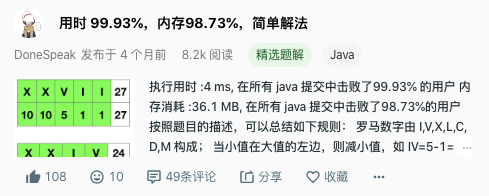LeetCode | 13. 罗马数字转整数DoneSpeak前言题目

• I 可以放在 V (5) 和 X (10) 的左边，来表示 4 和 9。

• X 可以放在 L (50) 和 C (100) 的左边，来表示 40 和 90。

• C 可以放在 D (500) 和 M (1000) 的左边，来表示 400 和 900。给定一个罗马数字，将其转换成整数。输入确保在 1 到 3999 的范围内。

解题

1. 罗马数字由 I,V,X,L,C,D,M 构成；

2. 当小值在大值的左边，则减小值，如IV=5-1=4

3. 当小值在大值的右边，则加小值，如VI=5+1=6

4. 由上可知，右值永远为正，因此最后一位必然为正。

import java.util.*;
class Solution { public int romanToInt(String s) { int sum = 0; int preNum = getValue(s.charAt(0)); for(int i = 1;i < s.length(); i ++) { int num = getValue(s.charAt(i)); if(preNum < num) { sum -= preNum; } else { sum += preNum; } preNum = num; } sum += preNum; return sum; } private int getValue(char ch) { switch(ch) { case 'I': return 1; case 'V': return 5; case 'X': return 10; case 'L': return 50; case 'C': return 100; case 'D': return 500; case 'M': return 1000; default: { // 这里也可以抛出异常，反正都不会跑到这里来 return 0 }; } }}DoneSpeak

Let the Work That I've Done Speak for Me 2018.05.10 加入

Java后端开发

评论